# How to calculate the length of service from hire date in Excel?

When it comes to working with dates, Excel may be a very helpful tool. You are able to perform computations based on dates thanks to the robust built-in formulas that this tool provides. The need to determine the number of years, months, or days that have passed since a pair of provided dates is a common example of a circumstance in which you could be required to make calculations with dates.

If you want to compute the number of years a person has worked for your organization and you know both the date they started and the date they left their position, this could be extremely helpful. The employee's resignation is a good time to inquire about the length of service they have provided.

It is possible that the goal is to ascertain the length of time that a person has been employed or to make use of that length of time in order to assess or compute any type of entitlement. If this is not the case, you may be trying to calculate the total number of years that the remaining employees have been with the company.

In this tutorial, we will take you through the process of calculating the number of years of service in terms of years, months, and days using various formulas and methodologies.

## Using YEARFRAC Function

You can use the YEARFRAC function if all you need to know is the total number of years of service that were accrued between two specific dates.

### Step 1

In our example we have some name of the employees with their joining date and end date of service in our excel sheet as shown in below given image. We are going to calculate the length of the service.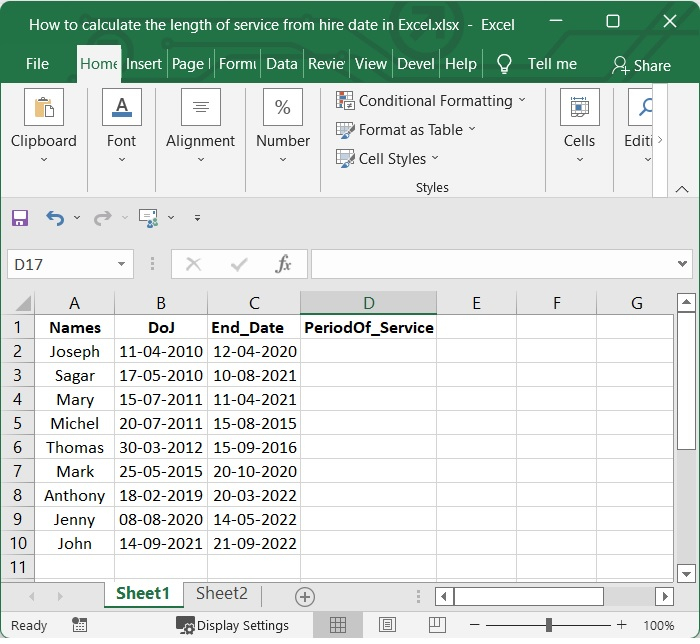### Step 2

Then select one blank cell and add the below given formula in it. In our example we have selected the cell D2 and added the below given formula in it.

=INT(YEARFRAC(B2,C2))


After adding the formula press enter to see the result.

In our example, B2 is the joining date cell and C2 is the end date cell. You can change the cell value as per your need. See the below given image.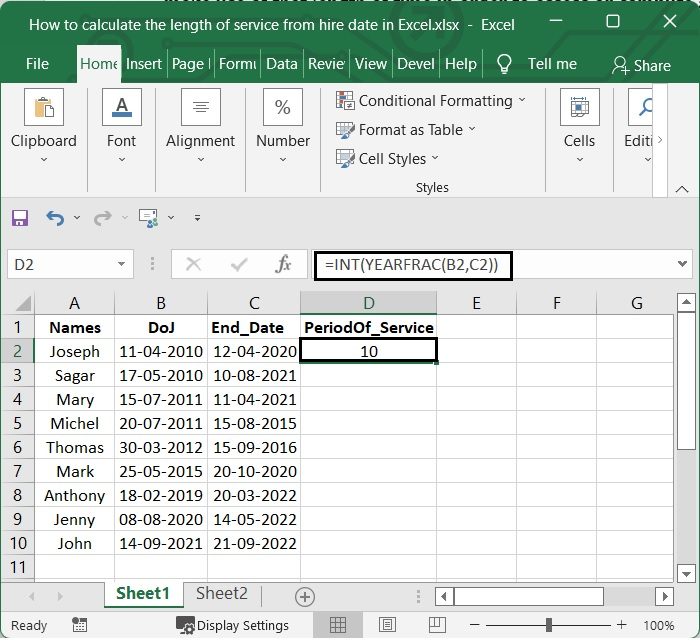### Step 3

Pull the auto fill handle all the way down to the cells where you want this formula to be applied. Check out this screenshot.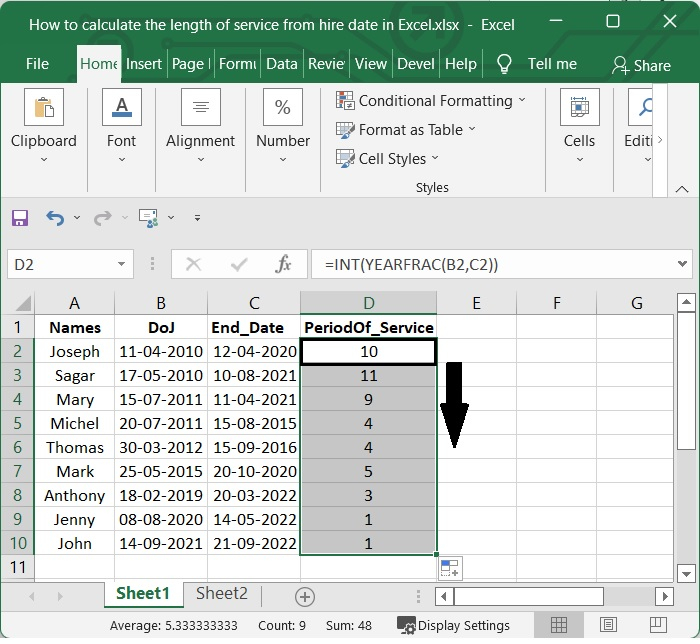## Using DATEDIF Function

Excel's DATEDIF function calculates the difference between two date values and returns the result in either years, months, or days. The DATEDIF function is referred to as a compatibility function.

The DATEDIF function can compute the time in years, months, or days that has elapsed between two specified dates (a start date and an end date). The unit argument, which is passed in as text, is used to provide the information necessary to specify the time unit.

### Step 1

Considering the above given example, if you want to know the service period of the employee using DATEDIF, add the below given formula to one blank cell.

=DATEDIF(B2, C2, "y")& " Years"


After adding the formula, press the Enter button to see the result. See the following screenshot.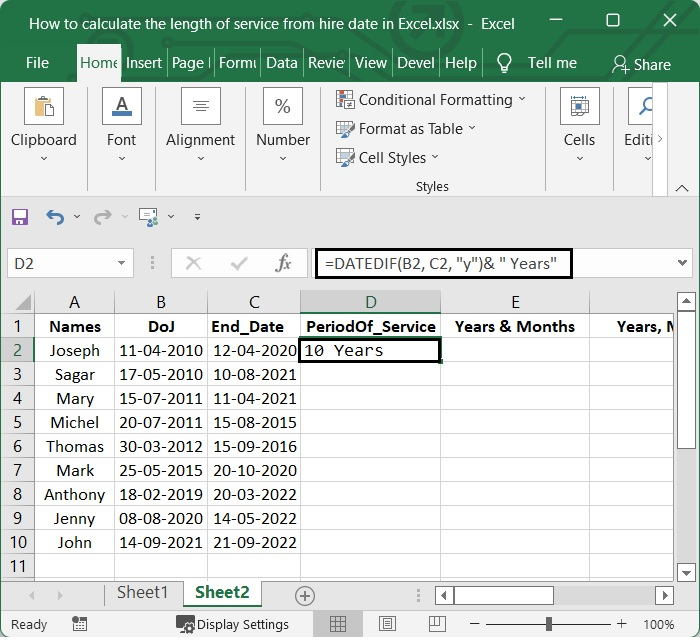### Step 2

Pull the auto fill handle all the way down to the cells where you want this formula to be applied. Check out this screenshot.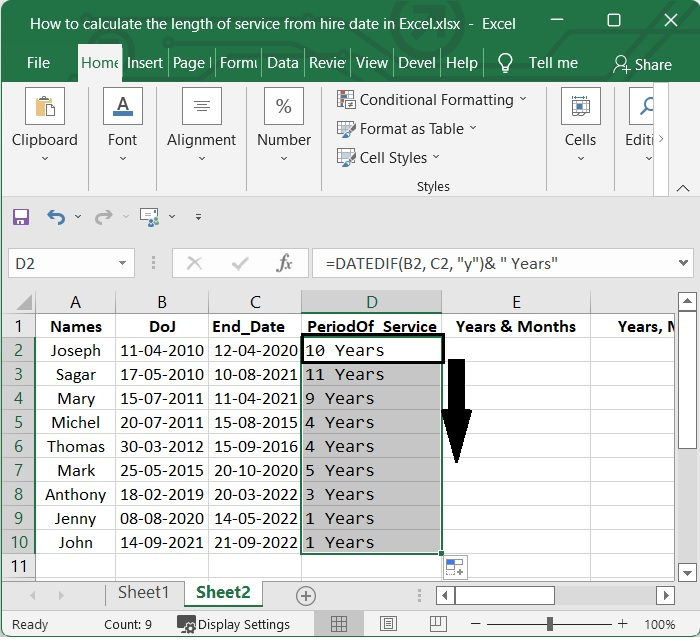## Calculating the Length of Service in Years and Months

Let's now find out how you can use Excel to calculate the length of service of an employee from the hire date.

### Step 1

Considering the above given example, if you want to know the service of the employee in years and months, add the below given formula to one blank cell.

=DATEDIF(B2,C2,"y")&" Years, "&DATEDIF(B2,C2,"ym")&" Months"


After adding the formula, press the Enter button to see the result. See the below given image.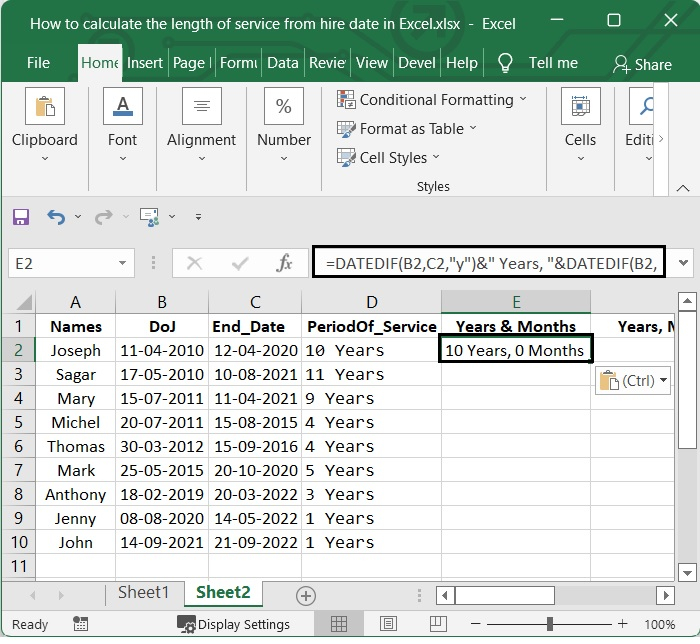### Step 2

Pull the auto-fill handle all the way down to the cells where you want this formula to be applied. Check out this screenshot.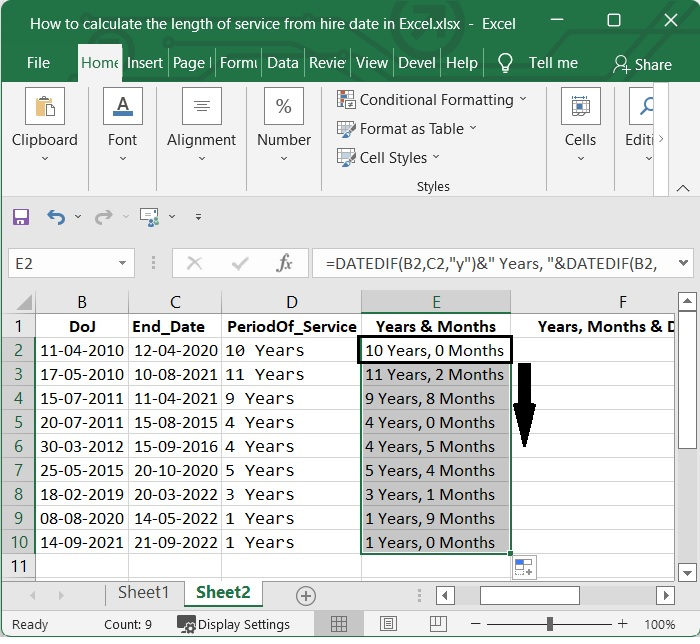## Calculating the Length of Service in Years, Months and Days

In this section, let's see how to you Excel to calculate the length of service in years, months, and days.

### Step 1

Considering the above given example, if you want to know the service of the employee in years, months and days, add the below given formula to one blank cell.

=DATEDIF(B2,C2,"y") & " Years, " & DATEDIF(B2,C2,"ym") & " Months, " & DATEDIF(B2,C2,"md") & " Days"


After adding the formula, press the Enter button to see the result. See the below given image.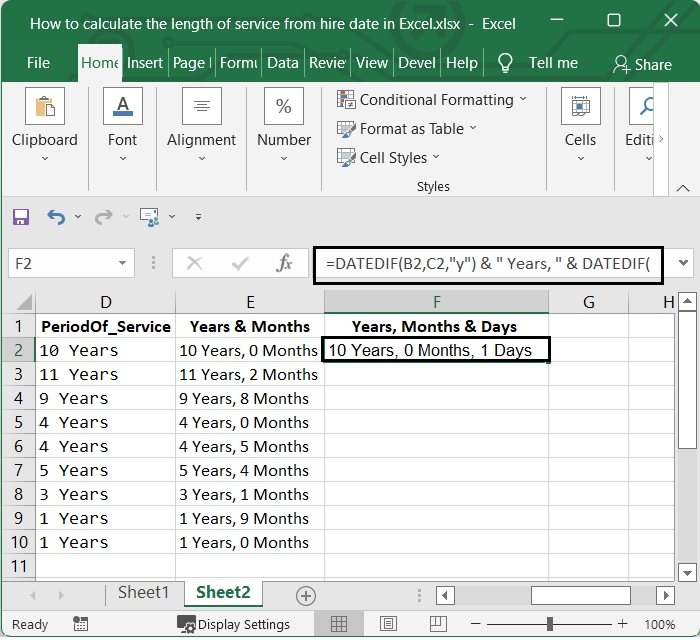### Step 2

Pull the auto fill handle all the way down to the cells where you want this formula to be applied. Check out this screenshot.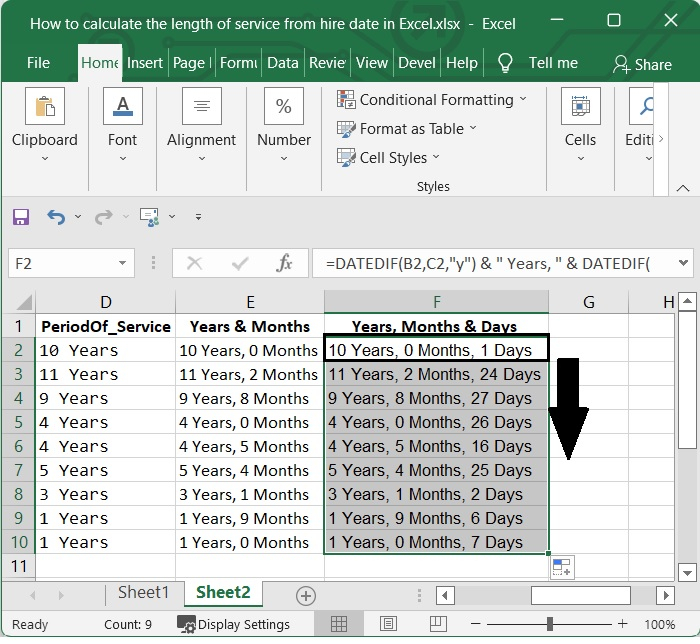## Conclusion

In this tutorial, we used a simple example to demonstrate how to use Excel to calculate the length of service using YEARFRAC function and DATEDIF function.